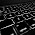## Thursday, 6 September 2012

### CBSE Class 10 - Maths - Ch1 - Real Numbers (MCQs)

Real NumbersSymbol to represent set of Real Numbers
(MCQs)

Q1: Which of the following has a non-terminating decimal expansion?

(a) 77/210
(b) 23/8
(c) 17/8
(d) 35/50

Q2(CBSE 2010): The decimal expansion of 141/120 will terminate after how many places of decimals?

(a) 1
(b) 2
(c) 3
(d) will not terminate

Q3: HCF of  84 and 270 is

(a) 8
(b) 6
(c) 4
(d) 2

Q4(CBSE 2010): If p, q are two consecutive natural numbers, then HCF(p, q) is:

(a) q
(b) p
(c) 1
(d) pq

Q5: If HCF if 60 and 168 is 12, what is the LCM

(a) 480
(b) 240
(c) 420
(d) 840

Q6(CBSE 2010): How many prime factors are there in prime factorisation of 5005 ?

(a) 2
(b) 4
(c) 6
(d) 7

Q7(CBSE 2010): A rational number can be expressed as a terminating decimal if the denominator has factors

(a) 2, 3 or 5
(b) 2 or 3
(c) 3 or 5
(d) 2 or 5

Q8(CBSE 2010): If p, q are two prime numbers, then LCM(p, q) is :

(a) 1
(b) p
(c) q
(d) pq

Q9: Let x = p/q be a rational number such that prime factorization of q is NOT in the form of 2n5m, where m and n are non-negative integers. Then x has a decimal representation which is:

(a) terminating
(b) Non-terminating, repeating
(c) Non-terminating, non-repeating
(d) None of these

Q10(CBSE 2010): Euclid’s division lemma states that for any two positive integer ‘a’ and ‘b’ there exists unique integers q and r such that a=bq+r where r must satisfy:

(a) 1 ≤  r  < b
(b) 0 < r ≤  b
(c) 0 ≤ r < b
(d) 0 < r < b

Q11(CBSE 2010): Which of the following is not an irrational number?

(a) 5 - √3
(b) 5 + √3
(c) 4 + √2
(d) 5 + √9

Q12(CBSE 2011): Which of the following numbers has terminating decimal expansion?

(a) 37/45
(b) 21/(2356)
(c) 17/49
(d) 89/(2232)

Q13: The mathematician who gave the term 'algorithm'?
(a) Euclid
(b) Gold Bach
(c) Khwarizmi
(d) Gauss

Q14: The decimal expansion of π
(a) is terminating.
(b) is non-terminating and repeating
(c) is non-terminating and non-repeating
(d) None of these

1: (a) 77/210
[Hint: For a rational number in the form p/q, such that the prime factors of q are not of the form 2n5m, where n and m are non-negative integers. Then the rational number has a decimal expansion which is non terminating repeating (recurring).]

2:  (c) 3 [Hint: Rational form of number p/q, where q = 2352]

3:  (b) 6

4:  (c) 1

5: (d) 840 [Hint: LCM × HCF = Product of two numbers]

6: (b) 4  [Hint: 5005 = 5×7×11×13]

7: (d) 2 or 5

8: (d) pq

9: (b) Non-terminating, repeating

10:  (c) 0 ≤ r < b

11:(d) 5 + √9 [Hint: √9 = 3, 5 + √9 = 8, a rational number]

12: (b) 21/(2356) [Hint: for rational number x = p/q, if factors are in the form of 2n5m, where n and m are non-negative integers, the rational number has a decimal expansion of terminating type.]

13: (c) Khwarizmi

14: (c) is non-terminating and non-repeating

See also CH1: Real Numbers (Study Points)
CH 1: Real Numbers (NCERT Ex 1.1)
CH 1: Real Numbers (Euclid's Division Lemma - Q & A)
CH 1: Problems on Euclid's Division Algorithm
CH 1: Real Numbers (Problems and Answers)
CH 1: Real Numbers (NCERT Ex 1.2)
CH 1: Real Numbers (NCERT Exemplar Ex 1.1 Q1-3)1.2.